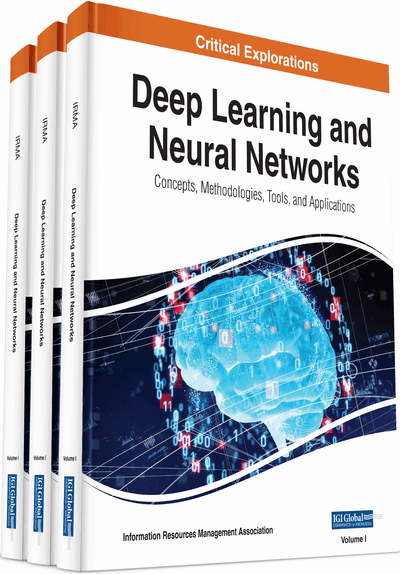# Prediction of Biosorption Capacity Using Artificial Neural Network Modeling and Genetic Algorithm: Prediction of Biosorption Capacity

Prakash Chandra Mishra, Anil Kumar Giri
DOI: 10.4018/978-1-7998-0414-7.ch010
OnDemand:
(Individual Chapters)
Available
\$37.50
No Current Special Offers

## Abstract

Artificial neural network model is applied for the prediction of the biosorption capacity of living cells of Bacillus cereus for the removal of chromium (VI) ions from aqueous solution. The maximum biosorption capacity of living cells of Bacillus cereus for chromium (VI) was found to be 89.24% at pH 7.5, equilibrium time of 60 min, biomass dosage of 6 g/L, and temperature of 30 ± 2 °C. The biosorption data of chromium (VI) ions collected from laboratory scale experimental set up is used to train a back propagation (BP) learning algorithm having 4-7-1 architecture. The model uses tangent sigmoid transfer function at input to hidden layer whereas a linear transfer function is used at output layer. The data is divided into training (75%) and testing (25%) sets. Comparison between the model results and experimental data gives a high degree of correlation R2 = 0.984 indicating that the model is able to predict the sorption efficiency with reasonable accuracy. Bacillus cereus biomass is characterized using AFM and FTIR.
Chapter Preview
Top

## 1. Introduction

ANNs are categorized as an artificial intelligence modeling technique due to their ability to recognize patterns and relationships in historical data and subsequently make inferences concerning new data. ANNs can be used for two broad categories of problems: data classification and parameter prediction. (1) Data classification problems, the ANN uses a specified algorithm to analyze data cases or patterns for similarities and then separates them into a pre-defined number of classes. (2) Parameter prediction problems, the ANN learns to predict accurately the value of an output parameter when information is given with sufficient input parameter (Yetilmezsoy and Demirel, 2008). The main applications of the ANN technique in the water treatment industry are in the development of process models and model-based process-control and automation tools.

### 1.1. Types of ANN Models

ANN models of drinking water treatment process can assume two distinct forms: (1) process models and (2) inverse process models. (1) The process model predicts the value of one or more process outputs, given the values of the process input parameters. An example of this type of model is the prediction of clarifier effluent turbidity using influent water parameters and operational parameters. (2) The inverse process model predicts the value of one or more process inputs, given the values of the remaining process inputs and process output(s). This type of model is often used to predict the value of an operational parameter required to reach a target effluent quality (Dimopoulos et al., 1999, Fernandes and Lona, 2005 and Gob et al., 1999).

### 1.2. Key Components of ANN Models

There are several key components to ANN models that are collectively referred to as the ANN architecture. Processing units or neurons perform primitive operations such as scaling data, summing weights, and amplifying or threshold sums. Neurons are organized into layers with each layer performing a specific function. The input layer serves as an interface between the input parameter data and the ANN model. Most models also contain one or two hidden layers, although more are possible (Aleboyeh et al., 2008; Belanche et al., 2008). These layers perform most of the iterative calculations within the network. The output layer serves as the interface between the ANN model and the end-user, transforming model information into an ANN-predicted value of the output parameter(s). Each neuron is connected to every neuron in adjacent layers by weights; links that represent the ‘strength’ of connection between neurons. Each ANN model has a propagation rule that defines how the weights connected to a neuron are combined to produce a net input. The propagation rule is generally a simple summation of the weights. As discussed, the input layer serves as an interface between input parameter data and the model. In this layer, a scaling function is used to scale data from their numeric range into a range that the network deals with efficiently, typically 0 to 1. The hidden and output layers contain an activation function that defines how the net input received by a neuron is combined with its current state of activation to produce a new state of activation. The most common activation function used in process modeling is the sigmoidal activation function. Finally, each network has a learning rule that defines how the weights are modified in order to minimize prediction error.

## Complete Chapter List

Search this Book:
Reset